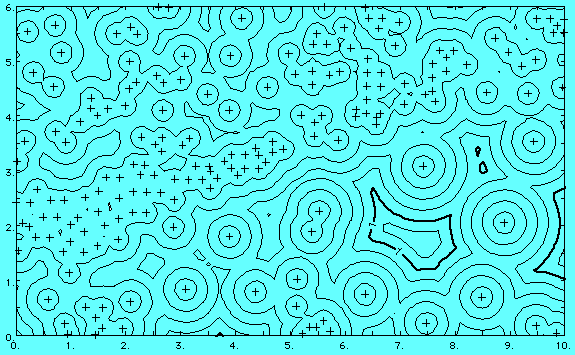## Distance function and similar maps

Global- and local-fit contouring procedures are appropriate for mapping surfaces that represent variables whose values are known at discrete observation points. In addition, Surface III will produce grids and maps that simply reflect the locations of the observation points themselves. They display distance functions which do not represent the values of a surface at all, but rather the distances from any location on the map to the locations of nearby control points. A distance function map is useful for assessing the reliability of a contour map made from the original data, as the contour map will obviously be of lower reliability in areas where control is most distant. Surface III can construct grids giving the distance to the nearest control point, the average distance to a specified number of control points, or the distance to the most distant of a specified number of points.

The following is an example of a typical distance function map. The contours show the distance from any point on the map to the nearest well (wells shown by plus symbols). The contour interval is 0.2 miles. Distance function maps also can be used to automatically define oil field boundaries, by drawing a single contour line a specified distance around all producing wells. Any phenomenon whose influence declines with distance may be analyzed by distance function maps.Spatial relationships between sample points can be analyzed using the Distance Map option of Surface III. Among other applications, it can be used to investigate the effectiveness of different search methods with specific data sets. Distance Map will display the local density of control points in the form of contour maps of the distance from each grid node to some specified set of control points.

Other statistics related to the local subset of observations around each grid node also can be computed and displayed as maps. These include maps of the distances to the nearest, farthest, and average neighbors of a point, the standard deviations of distances to neighbors of a point, the number of neighboring observations within specified distances of a point, the number of octants or quadrants containing specified numbers of points, and measures of the relative contributions of the nearest, farthest, and average neighbors to the estimates of values at the grid nodes.go back